[Code-Torch]

[Code-PyTorch]

[Code-Keras]

[Code-Caffe]

[Code-Tensorflow]

VGG-nets/ResNets： 堆叠相同形状的网络 building blocks；—— 网络 depth

Inceptions：split-transform-merge，将输入采用(1×1 Conv)分裂为几个低维 embedding，再经过一系列特定 filters (如3×3，5×5)的变换，最后连接在一起.

ResNeXt：采用 VGGs/ResNets 的网络的 depth 加深方式，同时利用 split-transform-merge 策略.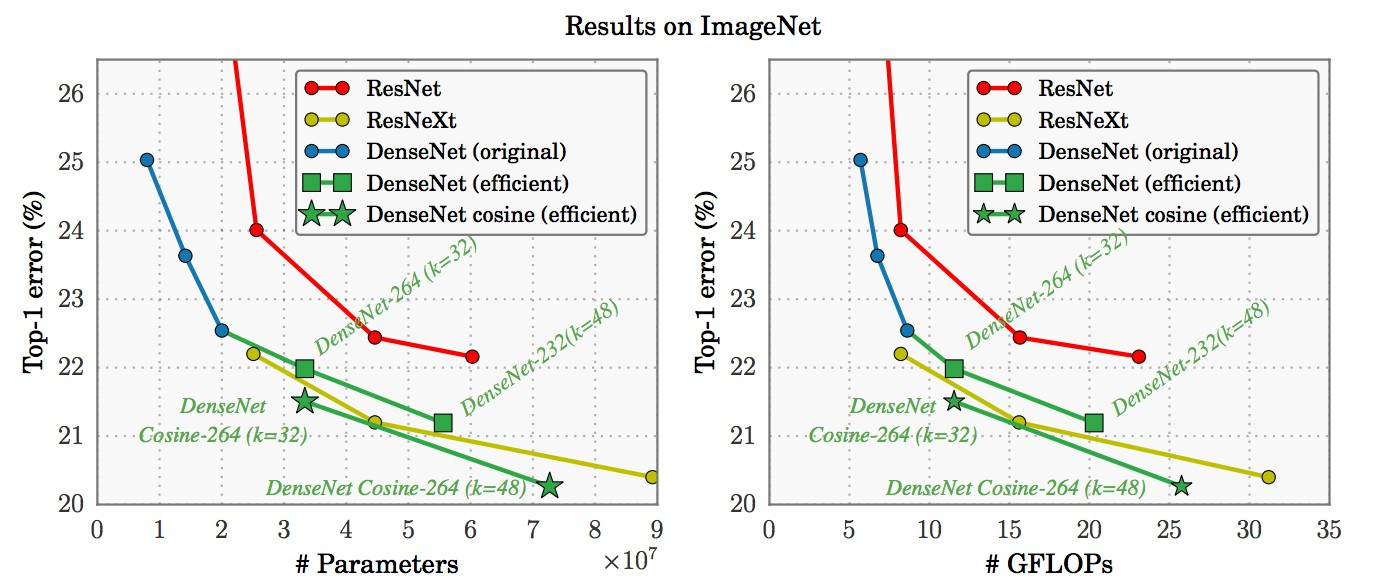<h2>1. Simple Neurons</h2>

ANN 中最简单的 neurons 是 inner product(内积)，其也可以看做是 aggregating transformation(聚合变换)：

${ \sum _{i=1}^D w_i x_i }$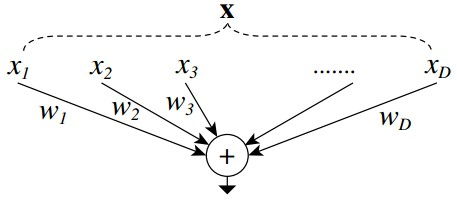<h2>2. Aggregated Transformations 聚合变换</h2>

aggregated transformations 表示为：

${ \mathcal{F} (\mathbf{x}) = \sum _{i=1}^C \mathcal{T} (\mathbf{x}) }$

${ C }$ 是待聚合的变换集的大小，即 Cardinality. 类似于 ${ D }$.

Cardinality 的维度决定了更复杂变换的数目.

${ \mathbf{y} = \mathbf{x} + \sum_{i=1}^C \mathcal{T}_i (\mathbf{x}) }$

${ \mathbf{y} }$ 为输出.

<h2>2.1 ResNet-50 && ResNeXt-50</h2>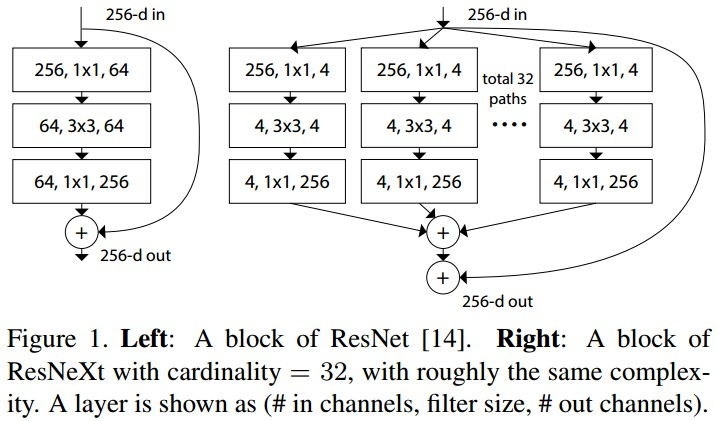Figure 1. Left - ResNet 的一个 block；Right - ResNeXt 的一个块，Cardinality=32. 二者复杂度相同.(#in_channels, #filter_size，#out_channels).

• 如果 spatial maps 是相同尺寸，blocks 共享相同的参数(width 和 filter sizes)
• 每次当 spatial map 基于因子 2 进行下采样时，blocks 的 width 乘上因子 2.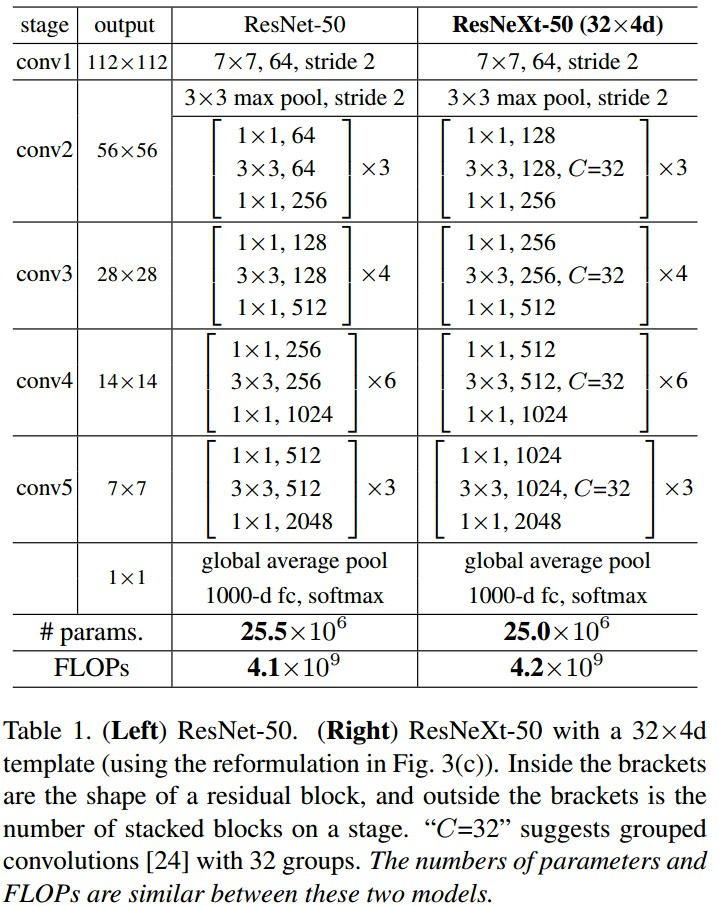ResNeXt 保留 ResNet 的堆叠 block，而是对单个 building block 改进.

<h3>2.2 ResNeXt && Inception-ResNet && Grouped Convolutions</h3>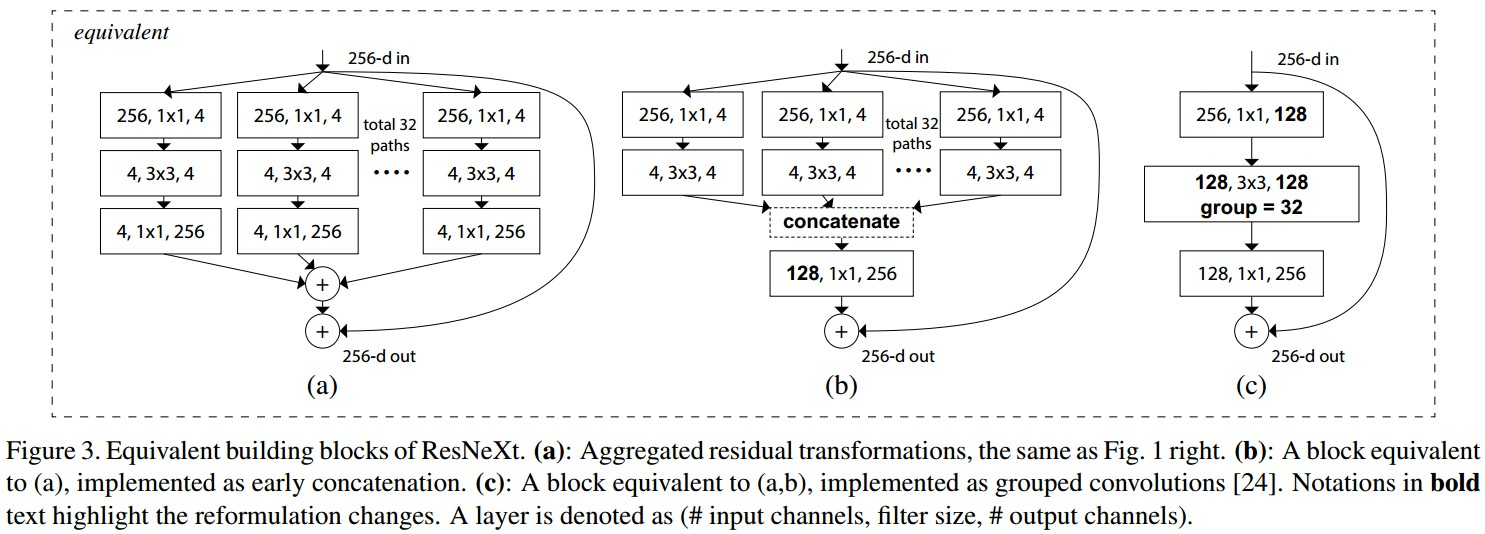Figure 3. ResNeXt building blocks 的等价形式. (a) Figure 1 Right 的 Aggregated transformations；(b) 采用提前进行加法操作的等价形式；(c) 采用 grouped conv 的等价形式.

Grouped Convolutions：group conv 层中，输入和输出的 channels 被分为 ${ C }$ 个 groups，分别对每个 group 进行 conv.

ResNeXt 只能在 block 的 depth>3时使用. 如果 block 的 depth=2，则会得到宽而密集的模块.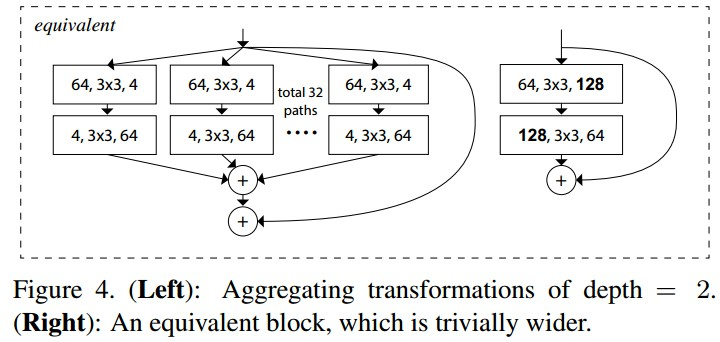<h3>2.3 参数</h3>

Figure 1 Left 中的 ResNet block参数：${ 256 \cdot 64 + 3 \cdot 3 \cdot 64 \cdot 64 + 64 \cdot 256 \approx 70K }$.

Figure 1 Right 中 ResNeXt block 参数：${ C \cdot (256 \cdot d + 3 \cdot 3 \cdot d \cdot d + d \cdot 256) }$. 当 C = 32，d=4 时，参数约有 70K.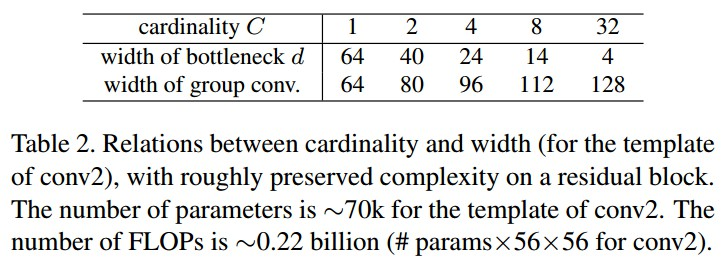<h2>3. Results</h2>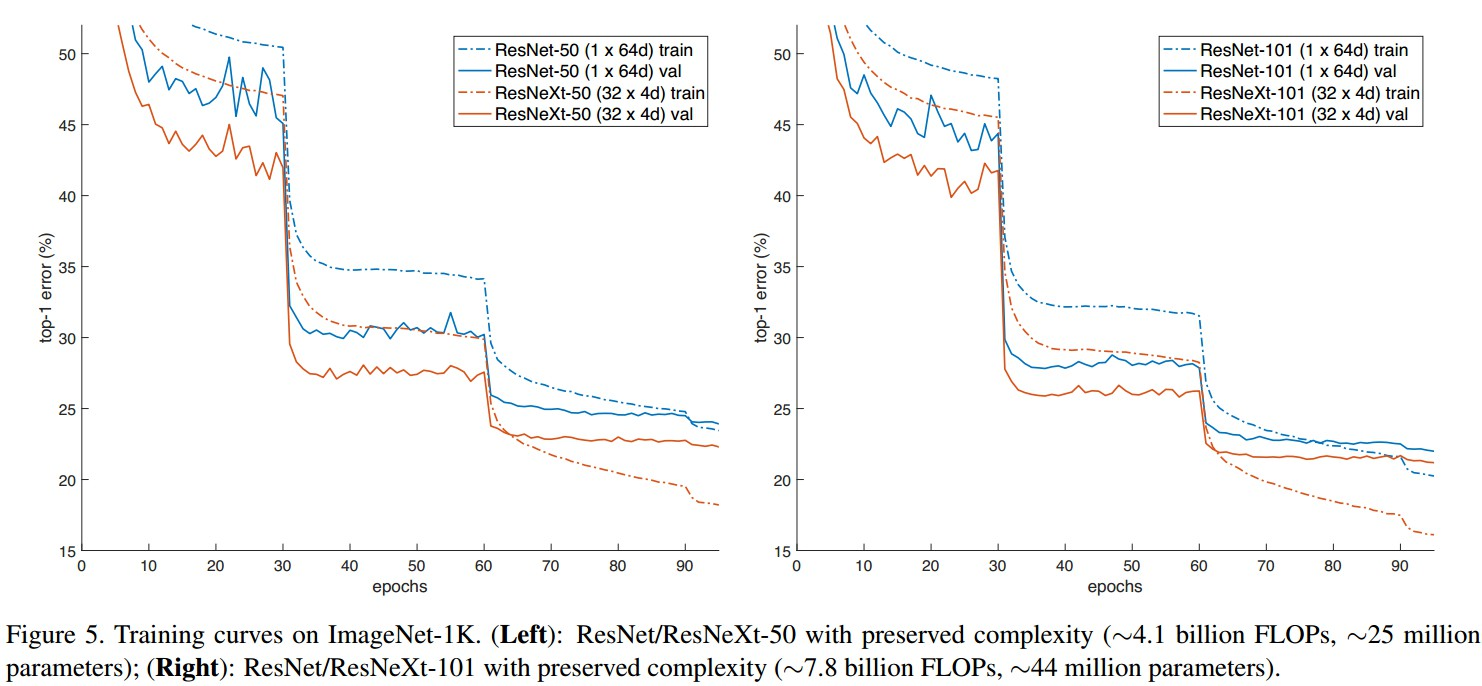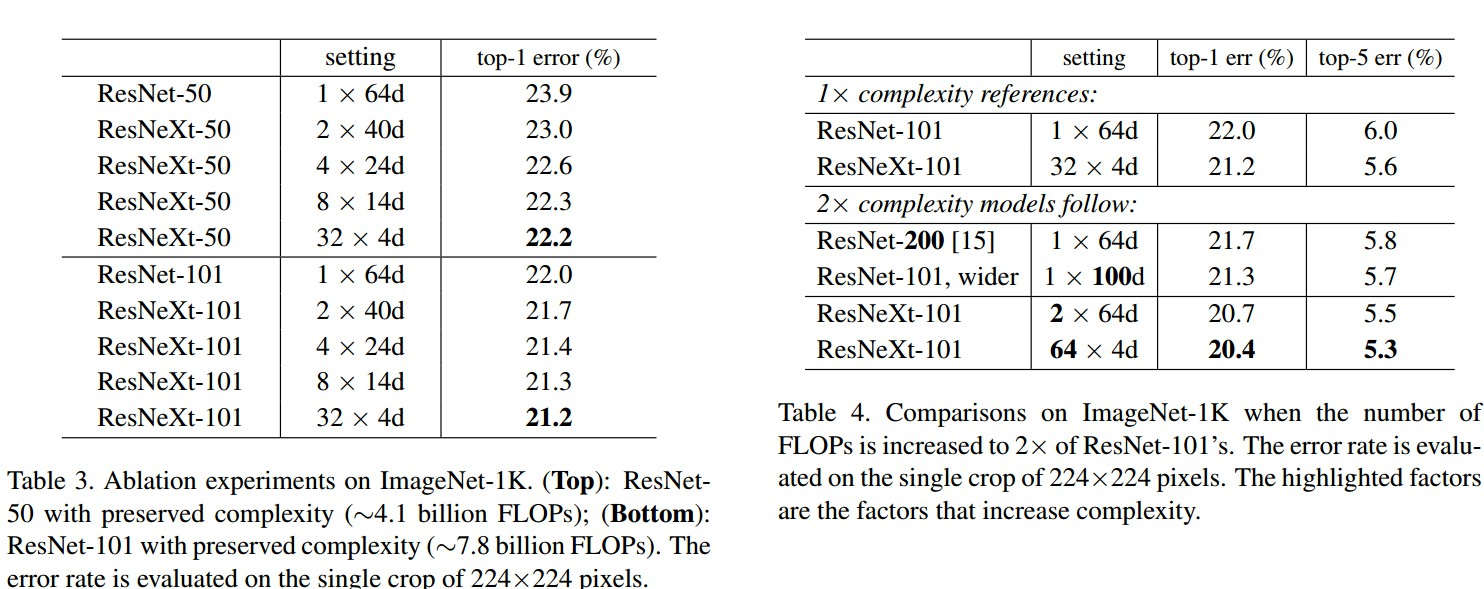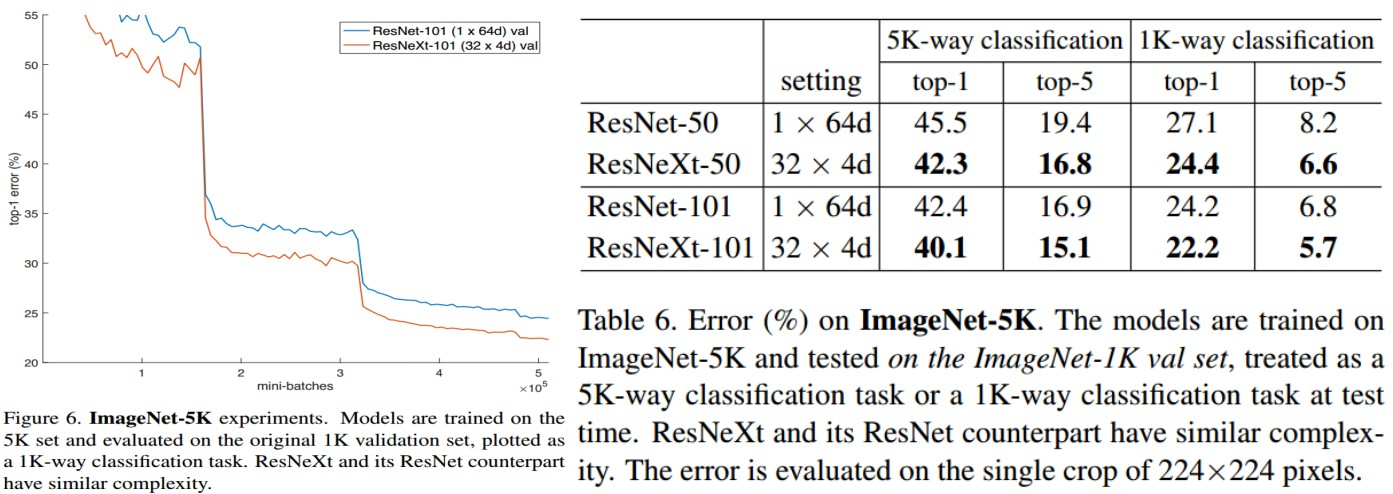<h2>4. 基于 ResNeXt 的 Faster R-CNN 实现</h2>

• 采用 Faster R-CNN框架；</p>
• 不共享 RPN 和 Fast R-CNN 的特征；
• RPN 阶段，在 8-GPUs 上训练，每个 GPU min-batch为 2 张图片，每张图片 256 个 anchors；
• RPN 训练阶段，前 120k mini-batches 的学习率为 learning_rate=0.02，后面 60K mini-batches 学习率为 learning_rate=0.002；
• Fast R-CNN 阶段，在 8-GPUs 上训练，每个 GPU 1张图片，每个 mini-batch 64 个regions；
• Fast R-CNN 训练阶段，前120k mini-batches 的学习率为 learning_rate=0.005，后面 60K mini-batches 学习率为 learning_rate=0.0005；
• weight_decay=0.0001，momentum=0.9.
• 其它实现类似于 Faster R-CNN.</p>

<h3>4.1 COCO 目标检测</h3>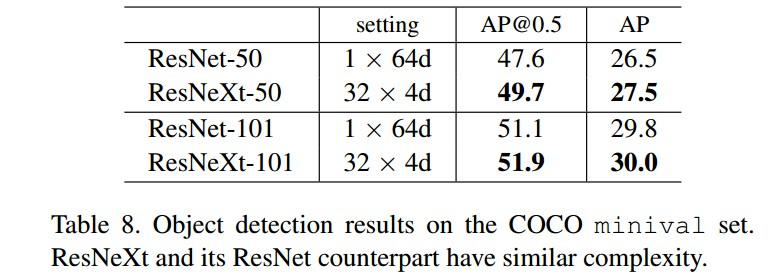<h2>5 Reference</h2>

 - ResNeXt算法详解
 - ResNext与Xception——对模型的新思考

Last modification：October 10th, 2018 at 01:56 am